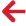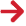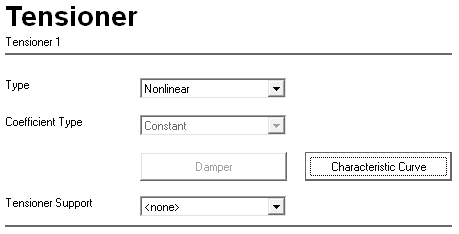﻿ PipeLay > Software Components & Operation > Defining a Vessel > Vessel Subcomponents > Tensioner

# TensionerThe Tensioner component is used to model the presence of a tensioner. The component is referenced in a similar manner to the Support component, via the Stinger Section, orcomponents.Tensioner Component

You can define a tensioner as a linear or nonlinear component. The default is a nonlinear Tensioner component. You can also optionally associate a tensioner support with a linear or nonlinear Tensioner component.

## non-linear tensioner

You can model this type of tensioner by setting the topmost drop-down box, Type, to Nonlinear. You can optionally specify a tensioner characteristic curve, in order to define the applied tension as function of tensioner payout. This allows you to simulate tensioner dead band and tensioner on the brake. The application of the characteristic curve relates to dynamic analyses only - in static analyses the tensioner is assumed to provide a constant restoring tension to restrain the pipeline in the static equilibrium configuration (which is typically governed by any installation criteria you specify in the Analysis component). Where several tensioners are included in a single static analysis, the restoring force is divided equally amongst them. To input characteristic curves, you click on the Characteristic Curve button to display the Tensioner Characteristic Curve dialog. Curves are defined as pairs of data points, in terms of normalised tension and displacement. The tension values are normalised with respect to the static equilibrium tension. By default, a constant tension is provided.

linear tensioner

You can model this type of tensioner by setting the topmost drop-down box, Type, to Linear. You have the option to specify a damping coefficient. This damping coefficient can either be constant or active type. To define a damping coefficient, you click on the Damping button to display the Tensioner Damper dialog. The layout of this dialog is dependent on the specified coefficient type however before going into detail on the dialog itself, it is worth noting a few basic points on the linear tensioner.

For a linear Tensioner component it is not possible to specify a characteristic curve. As a result, during a dynamic analysis, a constant restoring force is applied by the tensioner to the pipeline corresponding to the static tension. This means that the tensioner is free to extend and contract in response to the vessel motion during a dynamic analysis. However, due to the presence of damping, the velocity of the extensions and contractions can be limited. This, in turn, increases the tension variation of the pipeline at the location of the tensioner. The larger the damping coefficient value the greater the restriction on the extension/contraction velocities and so the greater the tension variation.

In the case of a constant linear tensioner there is a single input on the Tensioner Damper dialog, corresponding to the Damping Coefficient value. This coefficient input must always be a positive value and, as expected, it remains constant for the duration of an analysis.

For the linear active tensioner type, the Tensioner Damper dialog contains four inputs, the first of which is again the Damping Coefficient value. However in this case the coefficient value only represents an initial estimate, since it will be actively adjusted during a dynamic analysis so as to keep the tension applied by the tensioner between specified dead band limits. The default initial coefficient is a relatively high value of 100000 kN.s/m metric, or 6850 kips.s/ft imperial.

The dead band limits for the active type are specified through the second and third inputs on the dialog, which correspond to Maximum Tension Limit and Minimum Tension Limit. These inputs are percentage values of the static tension applied by the tensioner. The maximum limit must be a positive value while the minimum must be negative. The defaults correspond to plus and minus 10% respectively. This effectively means that the applied dynamic tension, by default, can vary between 90% and 110% of the static value.

In order to keep the applied dynamic tension within the limits discussed above, the following methodology is adopted for the active coefficient tensioner:

1.After each time step in the analysis the tension applied by the tensioner is checked to determine whether it is outside the specified limits. If this is the case, then a reduction of the damping coefficient is carried out, otherwise the analysis progresses as normal.

2.Once the reduction has been made the time step is solved for again and Step 1 is repeated. This process continues until the tension falls inside the problematic limit.

3.When the tension moves inside the limit a new check is performed to ensure that it hasn’t gone too far inside the limit. Note that this check requires a tolerance measure which comes directly from the fourth and final input on the Tensioner Damper dialog. This input is named Tension Tolerance and it defaults to 1%. If the new tension is inside the limit by a margin less than the specified tolerance then the analysis continues as normal, otherwise the coefficient must be increased appropriately.

4.If the coefficient is increased the time step is solved again and Steps 1 to 3 are repeated. This cycle continues until a desirable damping coefficient is obtained, that being a coefficient that keeps the tension within the permitted limits while at the same time allowing it to reach (get close to) the limits.

Note that the frequency at which it is necessary to perform the procedure above should decrease as an analysis progresses. This is due to the fact that the refined damping coefficient should gradually converge on an optimum value.